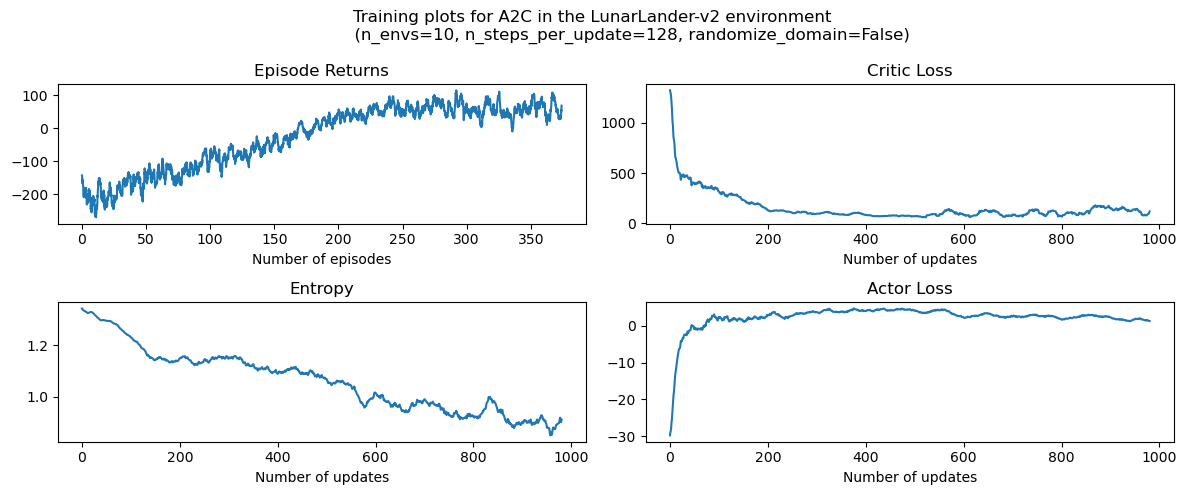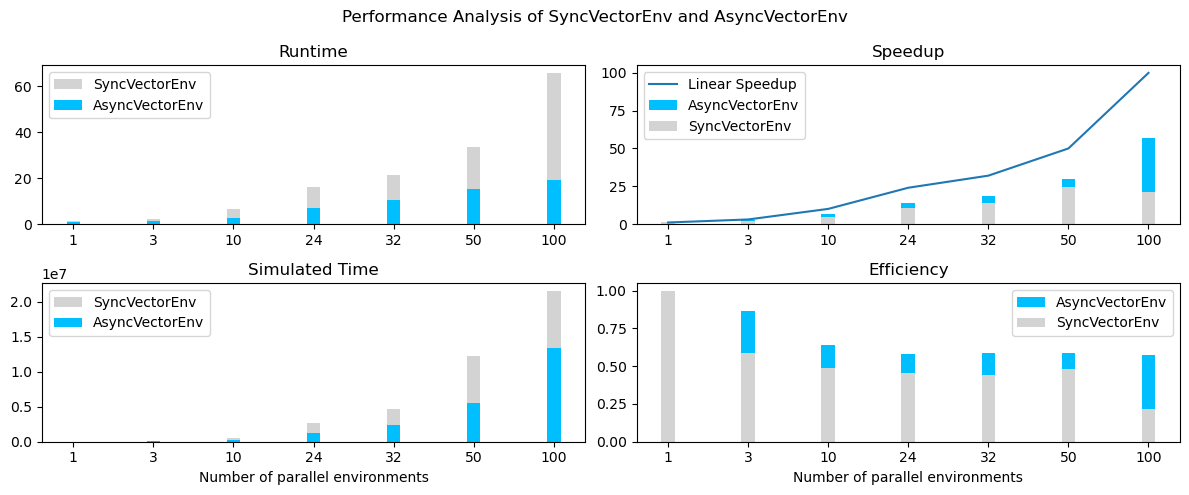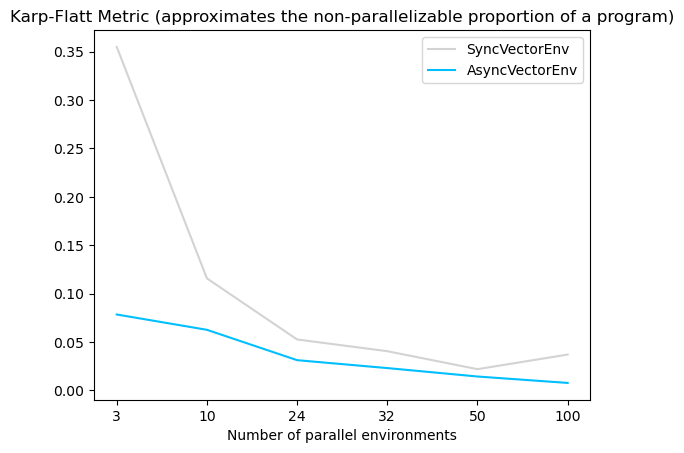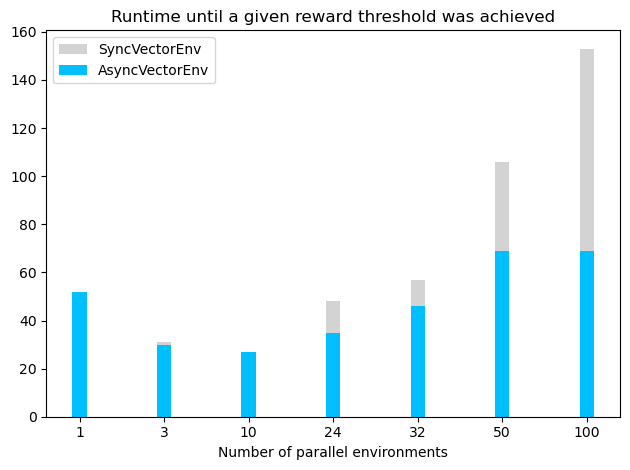# Training A2C with Vector Envs and Domain Randomization#

## Notice#

If you encounter an RuntimeError like the following comment raised on multiprocessing/spawn.py, wrap up the code from `gym.vector.make=` or `gym.vector.AsyncVectorEnv` to the end of the code by `if__name__ == '__main__'`.

`An attempt has been made to start a new process before the current process has finished its bootstrapping phase.`

## Introduction#

In this tutorial, you’ll learn how to use vectorized environments to train an Advantage Actor-Critic agent. We are going to use A2C, which is the synchronous version of the A3C algorithm .

Vectorized environments  can help to achieve quicker and more robust training by allowing multiple instances of the same environment to run in parallel (on multiple CPUs). This can significantly reduce the variance and thus speeds up the training.

We will implement an Advantage Actor-Critic from scratch to look at how you can feed batched states into your networks to get a vector of actions (one action per environment) and calculate the losses for actor and critic on minibatches of transitions. Each minibatch contains the transitions of one sampling phase: n_steps_per_update steps are executed in n_envs environments in parallel (multiply the two to get the number of transitions in a minibatch). After each sampling phase, the losses are calculated and one gradient step is executed. To calculate the advantages, we are going to use the Generalized Advantage Estimation (GAE) method , which balances the tradeoff between variance and bias of the advantage estimates.

The A2C agent class is initialized with the number of features of the input state, the number of actions the agent can take, the learning rates and the number of environments that run in parallel to collect experiences. The actor and critic networks are defined and their respective optimizers are initialized. The forward pass of the networks takes in a batched vector of states and returns a tensor of state values and a tensor of action logits. The select_action method returns a tuple of the chosen actions, the log-probs of those actions, and the state values for each action. In addition, it also returns the entropy of the policy distribution, which is subtracted from the loss later (with a weighting factor ent_coef) to encourage exploration.

The get_losses function calculates the losses for the actor and critic networks (using GAE), which are then updated using the update_parameters function.

```# Author: Till Zemann

from __future__ import annotations

import os

import matplotlib.pyplot as plt
import numpy as np
import torch
import torch.nn as nn
from torch import optim
from tqdm import tqdm

import gymnasium as gym
```

The Actor-Critic combines elements of value-based and policy-based methods. In A2C, the agent has two separate neural networks: a critic network that estimates the state-value function, and an actor network that outputs logits for a categorical probability distribution over all actions. The critic network is trained to minimize the mean squared error between the predicted state values and the actual returns received by the agent (this is equivalent to minimizing the squared advantages, because the advantage of an action is as the difference between the return and the state-value: A(s,a) = Q(s,a) - V(s). The actor network is trained to maximize the expected return by selecting actions that have high expected values according to the critic network.

The focus of this tutorial will not be on the details of A2C itself. Instead, the tutorial will focus on how to use vectorized environments and domain randomization to accelerate the training process for A2C (and other reinforcement learning algorithms).

```class A2C(nn.Module):
"""

Args:
n_features: The number of features of the input state.
n_actions: The number of actions the agent can take.
device: The device to run the computations on (running on a GPU might be quicker for larger Neural Nets,
for this code CPU is totally fine).
critic_lr: The learning rate for the critic network (should usually be larger than the actor_lr).
actor_lr: The learning rate for the actor network.
n_envs: The number of environments that run in parallel (on multiple CPUs) to collect experiences.
"""

def __init__(
self,
n_features: int,
n_actions: int,
device: torch.device,
critic_lr: float,
actor_lr: float,
n_envs: int,
) -> None:
"""Initializes the actor and critic networks and their respective optimizers."""
super().__init__()
self.device = device
self.n_envs = n_envs

critic_layers = [
nn.Linear(n_features, 32),
nn.ReLU(),
nn.Linear(32, 32),
nn.ReLU(),
nn.Linear(32, 1),  # estimate V(s)
]

actor_layers = [
nn.Linear(n_features, 32),
nn.ReLU(),
nn.Linear(32, 32),
nn.ReLU(),
nn.Linear(
32, n_actions
),  # estimate action logits (will be fed into a softmax later)
]

# define actor and critic networks
self.critic = nn.Sequential(*critic_layers).to(self.device)
self.actor = nn.Sequential(*actor_layers).to(self.device)

# define optimizers for actor and critic
self.critic_optim = optim.RMSprop(self.critic.parameters(), lr=critic_lr)
self.actor_optim = optim.RMSprop(self.actor.parameters(), lr=actor_lr)

def forward(self, x: np.ndarray) -> tuple[torch.Tensor, torch.Tensor]:
"""
Forward pass of the networks.

Args:
x: A batched vector of states.

Returns:
state_values: A tensor with the state values, with shape [n_envs,].
action_logits_vec: A tensor with the action logits, with shape [n_envs, n_actions].
"""
x = torch.Tensor(x).to(self.device)
state_values = self.critic(x)  # shape: [n_envs,]
action_logits_vec = self.actor(x)  # shape: [n_envs, n_actions]
return (state_values, action_logits_vec)

def select_action(
self, x: np.ndarray
) -> tuple[torch.Tensor, torch.Tensor, torch.Tensor, torch.Tensor]:
"""
Returns a tuple of the chosen actions and the log-probs of those actions.

Args:
x: A batched vector of states.

Returns:
actions: A tensor with the actions, with shape [n_steps_per_update, n_envs].
action_log_probs: A tensor with the log-probs of the actions, with shape [n_steps_per_update, n_envs].
state_values: A tensor with the state values, with shape [n_steps_per_update, n_envs].
"""
state_values, action_logits = self.forward(x)
action_pd = torch.distributions.Categorical(
logits=action_logits
)  # implicitly uses softmax
actions = action_pd.sample()
action_log_probs = action_pd.log_prob(actions)
entropy = action_pd.entropy()
return (actions, action_log_probs, state_values, entropy)

def get_losses(
self,
rewards: torch.Tensor,
action_log_probs: torch.Tensor,
value_preds: torch.Tensor,
entropy: torch.Tensor,
gamma: float,
lam: float,
ent_coef: float,
device: torch.device,
) -> tuple[torch.Tensor, torch.Tensor]:
"""
Computes the loss of a minibatch (transitions collected in one sampling phase) for actor and critic

Args:
rewards: A tensor with the rewards for each time step in the episode, with shape [n_steps_per_update, n_envs].
action_log_probs: A tensor with the log-probs of the actions taken at each time step in the episode, with shape [n_steps_per_update, n_envs].
value_preds: A tensor with the state value predictions for each time step in the episode, with shape [n_steps_per_update, n_envs].
masks: A tensor with the masks for each time step in the episode, with shape [n_steps_per_update, n_envs].
gamma: The discount factor.
lam: The GAE hyperparameter. (lam=1 corresponds to Monte-Carlo sampling with high variance and no bias,
and lam=0 corresponds to normal TD-Learning that has a low variance but is biased
because the estimates are generated by a Neural Net).
device: The device to run the computations on (e.g. CPU or GPU).

Returns:
critic_loss: The critic loss for the minibatch.
actor_loss: The actor loss for the minibatch.
"""
T = len(rewards)

# compute the advantages using GAE
gae = 0.0
for t in reversed(range(T - 1)):
td_error = (
rewards[t] + gamma * masks[t] * value_preds[t + 1] - value_preds[t]
)
gae = td_error + gamma * lam * masks[t] * gae

# calculate the loss of the minibatch for actor and critic

# give a bonus for higher entropy to encourage exploration
actor_loss = (
-(advantages.detach() * action_log_probs).mean() - ent_coef * entropy.mean()
)
return (critic_loss, actor_loss)

def update_parameters(
self, critic_loss: torch.Tensor, actor_loss: torch.Tensor
) -> None:
"""
Updates the parameters of the actor and critic networks.

Args:
critic_loss: The critic loss.
actor_loss: The actor loss.
"""
critic_loss.backward()
self.critic_optim.step()

actor_loss.backward()
self.actor_optim.step()
```

## Using Vectorized Environments#

When you calculate the losses for the two Neural Networks over only one epoch, it might have a high variance. With vectorized environments, we can play with n_envs in parallel and thus get up to a linear speedup (meaning that in theory, we collect samples n_envs times quicker) that we can use to calculate the loss for the current policy and critic network. When we are using more samples to calculate the loss, it will have a lower variance and theirfore leads to quicker learning.

A2C is a synchronous method, meaning that the parameter updates to Networks take place deterministically (after each sampling phase), but we can still make use of asynchronous vector envs to spawn multiple processes for parallel environment execution.

The simplest way to create vector environments is by calling gym.vector.make, which creates multiple instances of the same environment:

```envs = gym.vector.make("LunarLander-v2", num_envs=3, max_episode_steps=600)
```

## Domain Randomization#

If we want to randomize the environment for training to get more robust agents (that can deal with different parameterizations of an environment and theirfore might have a higher degree of generalization), we can set the desired parameters manually or use a pseudo-random number generator to generate them.

Manually setting up 3 parallel ‘LunarLander-v2’ envs with different parameters:

```envs = gym.vector.AsyncVectorEnv(
[
lambda: gym.make(
"LunarLander-v2",
gravity=-10.0,
enable_wind=True,
wind_power=15.0,
turbulence_power=1.5,
max_episode_steps=600,
),
lambda: gym.make(
"LunarLander-v2",
gravity=-9.8,
enable_wind=True,
wind_power=10.0,
turbulence_power=1.3,
max_episode_steps=600,
),
lambda: gym.make(
"LunarLander-v2", gravity=-7.0, enable_wind=False, max_episode_steps=600
),
]
)
```

Randomly generating the parameters for 3 parallel ‘LunarLander-v2’ envs, using np.clip to stay in the recommended parameter space:

```envs = gym.vector.AsyncVectorEnv(
[
lambda: gym.make(
"LunarLander-v2",
gravity=np.clip(
np.random.normal(loc=-10.0, scale=1.0), a_min=-11.99, a_max=-0.01
),
enable_wind=np.random.choice([True, False]),
wind_power=np.clip(
np.random.normal(loc=15.0, scale=1.0), a_min=0.01, a_max=19.99
),
turbulence_power=np.clip(
np.random.normal(loc=1.5, scale=0.5), a_min=0.01, a_max=1.99
),
max_episode_steps=600,
)
for i in range(3)
]
)
```

Here we are using normal distributions with the standard parameterization of the environment as the mean and an arbitrary standard deviation (scale). Depending on the problem, you can experiment with higher variance and use different distributions as well.

If you are training on the same n_envs environments for the entire training time, and n_envs is a relatively low number (in proportion to how complex the environment is), you might still get some overfitting to the specific parameterizations that you picked. To mitigate this, you can either pick a high number of randomly parameterized environments or remake your environments every couple of sampling phases to generate a new set of pseudo-random parameters.

## Setup#

```# environment hyperparams
n_envs = 10
n_steps_per_update = 128
randomize_domain = False

# agent hyperparams
gamma = 0.999
lam = 0.95  # hyperparameter for GAE
ent_coef = 0.01  # coefficient for the entropy bonus (to encourage exploration)
actor_lr = 0.001
critic_lr = 0.005

# Note: the actor has a slower learning rate so that the value targets become
# more stationary and are theirfore easier to estimate for the critic

# environment setup
if randomize_domain:
envs = gym.vector.AsyncVectorEnv(
[
lambda: gym.make(
"LunarLander-v2",
gravity=np.clip(
np.random.normal(loc=-10.0, scale=1.0), a_min=-11.99, a_max=-0.01
),
enable_wind=np.random.choice([True, False]),
wind_power=np.clip(
np.random.normal(loc=15.0, scale=1.0), a_min=0.01, a_max=19.99
),
turbulence_power=np.clip(
np.random.normal(loc=1.5, scale=0.5), a_min=0.01, a_max=1.99
),
max_episode_steps=600,
)
for i in range(n_envs)
]
)

else:
envs = gym.vector.make("LunarLander-v2", num_envs=n_envs, max_episode_steps=600)

obs_shape = envs.single_observation_space.shape
action_shape = envs.single_action_space.n

# set the device
use_cuda = False
if use_cuda:
device = torch.device("cuda" if torch.cuda.is_available() else "cpu")
else:
device = torch.device("cpu")

# init the agent
agent = A2C(obs_shape, action_shape, device, critic_lr, actor_lr, n_envs)
```

## Training the A2C Agent#

For our training loop, we are using the RecordEpisodeStatistics wrapper to record the episode lengths and returns and we are also saving the losses and entropies to plot them after the agent finished training.

You may notice that the don’t reset the vectorized envs at the start of each episode like we would usually do. This is because each environment resets automatically once the episode finishes (each environment takes a different number of timesteps to finish an episode because of the random seeds). As a result, we are also not collecting data in episodes, but rather just play a certain number of steps (n_steps_per_update) in each environment (as an example, this could mean that we play 20 timesteps to finish an episode and then use the rest of the timesteps to begin a new one).

```# create a wrapper environment to save episode returns and episode lengths
envs_wrapper = gym.wrappers.RecordEpisodeStatistics(envs, deque_size=n_envs * n_updates)

critic_losses = []
actor_losses = []
entropies = []

# use tqdm to get a progress bar for training
# we don't have to reset the envs, they just continue playing
# until the episode is over and then reset automatically

# reset lists that collect experiences of an episode (sample phase)
ep_value_preds = torch.zeros(n_steps_per_update, n_envs, device=device)
ep_rewards = torch.zeros(n_steps_per_update, n_envs, device=device)
ep_action_log_probs = torch.zeros(n_steps_per_update, n_envs, device=device)

# at the start of training reset all envs to get an initial state
if sample_phase == 0:
states, info = envs_wrapper.reset(seed=42)

# play n steps in our parallel environments to collect data
for step in range(n_steps_per_update):
# select an action A_{t} using S_{t} as input for the agent
actions, action_log_probs, state_value_preds, entropy = agent.select_action(
states
)

# perform the action A_{t} in the environment to get S_{t+1} and R_{t+1}
states, rewards, terminated, truncated, infos = envs_wrapper.step(
actions.cpu().numpy()
)

ep_value_preds[step] = torch.squeeze(state_value_preds)
ep_rewards[step] = torch.tensor(rewards, device=device)
ep_action_log_probs[step] = action_log_probs

# for each env the mask is 1 if the episode is ongoing and 0 if it is terminated (not by truncation!)
masks[step] = torch.tensor([not term for term in terminated])

# calculate the losses for actor and critic
critic_loss, actor_loss = agent.get_losses(
ep_rewards,
ep_action_log_probs,
ep_value_preds,
entropy,
gamma,
lam,
ent_coef,
device,
)

# update the actor and critic networks
agent.update_parameters(critic_loss, actor_loss)

# log the losses and entropy
critic_losses.append(critic_loss.detach().cpu().numpy())
actor_losses.append(actor_loss.detach().cpu().numpy())
entropies.append(entropy.detach().mean().cpu().numpy())
```

## Plotting#

```""" plot the results """

# %matplotlib inline

rolling_length = 20
fig, axs = plt.subplots(nrows=2, ncols=2, figsize=(12, 5))
fig.suptitle(
f"Training plots for {agent.__class__.__name__} in the LunarLander-v2 environment \n \
(n_envs={n_envs}, n_steps_per_update={n_steps_per_update}, randomize_domain={randomize_domain})"
)

# episode return
axs.set_title("Episode Returns")
episode_returns_moving_average = (
np.convolve(
np.array(envs_wrapper.return_queue).flatten(),
np.ones(rolling_length),
mode="valid",
)
/ rolling_length
)
axs.plot(
np.arange(len(episode_returns_moving_average)) / n_envs,
episode_returns_moving_average,
)
axs.set_xlabel("Number of episodes")

# entropy
axs.set_title("Entropy")
entropy_moving_average = (
np.convolve(np.array(entropies), np.ones(rolling_length), mode="valid")
/ rolling_length
)
axs.plot(entropy_moving_average)

# critic loss
axs.set_title("Critic Loss")
critic_losses_moving_average = (
np.convolve(
np.array(critic_losses).flatten(), np.ones(rolling_length), mode="valid"
)
/ rolling_length
)
axs.plot(critic_losses_moving_average)

# actor loss
axs.set_title("Actor Loss")
actor_losses_moving_average = (
np.convolve(np.array(actor_losses).flatten(), np.ones(rolling_length), mode="valid")
/ rolling_length
)
axs.plot(actor_losses_moving_average)

plt.tight_layout()
plt.show()
```## Performance Analysis of Synchronous and Asynchronous Vectorized Environments#

Asynchronous environments can lead to quicker training times and a higher speedup for data collection compared to synchronous environments. This is because asynchronous environments allow multiple agents to interact with their environments in parallel, while synchronous environments run multiple environments serially. This results in better efficiency and faster training times for asynchronous environments.According to the Karp-Flatt metric (a metric used in parallel computing to estimate the limit for the speedup when scaling up the number of parallel processes, here the number of environments), the estimated max. speedup for asynchronous environments is 57, while the estimated maximum speedup for synchronous environments is 21. This suggests that asynchronous environments have significantly faster training times compared to synchronous environments (see graphs).However, it is important to note that increasing the number of parallel vector environments can lead to slower training times after a certain number of environments (see plot below, where the agent was trained until the mean training returns were above -120). The slower training times might occur because the gradients of the environments are good enough after a relatively low number of environments (especially if the environment is not very complex). In this case, increasing the number of environments does not increase the learning speed, and actually increases the runtime, possibly due to the additional time needed to calculate the gradients. For LunarLander-v2, the best performing configuration used a AsyncVectorEnv with 10 parallel environments, but environments with a higher complexity may require more parallel environments to achieve optimal performance.```save_weights = False

actor_weights_path = "weights/actor_weights.h5"
critic_weights_path = "weights/critic_weights.h5"

if not os.path.exists("weights"):
os.mkdir("weights")

""" save network weights """
if save_weights:
torch.save(agent.actor.state_dict(), actor_weights_path)
torch.save(agent.critic.state_dict(), critic_weights_path)

agent = A2C(obs_shape, action_shape, device, critic_lr, actor_lr)

agent.actor.eval()
agent.critic.eval()
```

## Showcase the Agent#

```""" play a couple of showcase episodes """

n_showcase_episodes = 3

for episode in range(n_showcase_episodes):
print(f"starting episode {episode}...")

# create a new sample environment to get new random parameters
if randomize_domain:
env = gym.make(
"LunarLander-v2",
render_mode="human",
gravity=np.clip(
np.random.normal(loc=-10.0, scale=2.0), a_min=-11.99, a_max=-0.01
),
enable_wind=np.random.choice([True, False]),
wind_power=np.clip(
np.random.normal(loc=15.0, scale=2.0), a_min=0.01, a_max=19.99
),
turbulence_power=np.clip(
np.random.normal(loc=1.5, scale=1.0), a_min=0.01, a_max=1.99
),
max_episode_steps=500,
)
else:
env = gym.make("LunarLander-v2", render_mode="human", max_episode_steps=500)

# get an initial state
state, info = env.reset()

# play one episode
done = False
while not done:
# select an action A_{t} using S_{t} as input for the agent
action, _, _, _ = agent.select_action(state[None, :])

# perform the action A_{t} in the environment to get S_{t+1} and R_{t+1}
state, reward, terminated, truncated, info = env.step(action.item())

# update if the environment is done
done = terminated or truncated

env.close()
```

## Try playing the environment yourself#

```# from gymnasium.utils.play import play
#
# play(gym.make('LunarLander-v2', render_mode='rgb_array'),
#     keys_to_action={'w': 2, 'a': 1, 'd': 3}, noop=0)
```